/* styles */ We know wine and water don't mix, but Italian olive oil and wine make a perfect combo! Grad your loaf of bread, order tour bottles and join Regine T. Rousseau, the Shall We Wine Tribe, Vero, and La Maliosa on April 30, 2021 @ 6pm CST as we explore Italian wines and olive oils.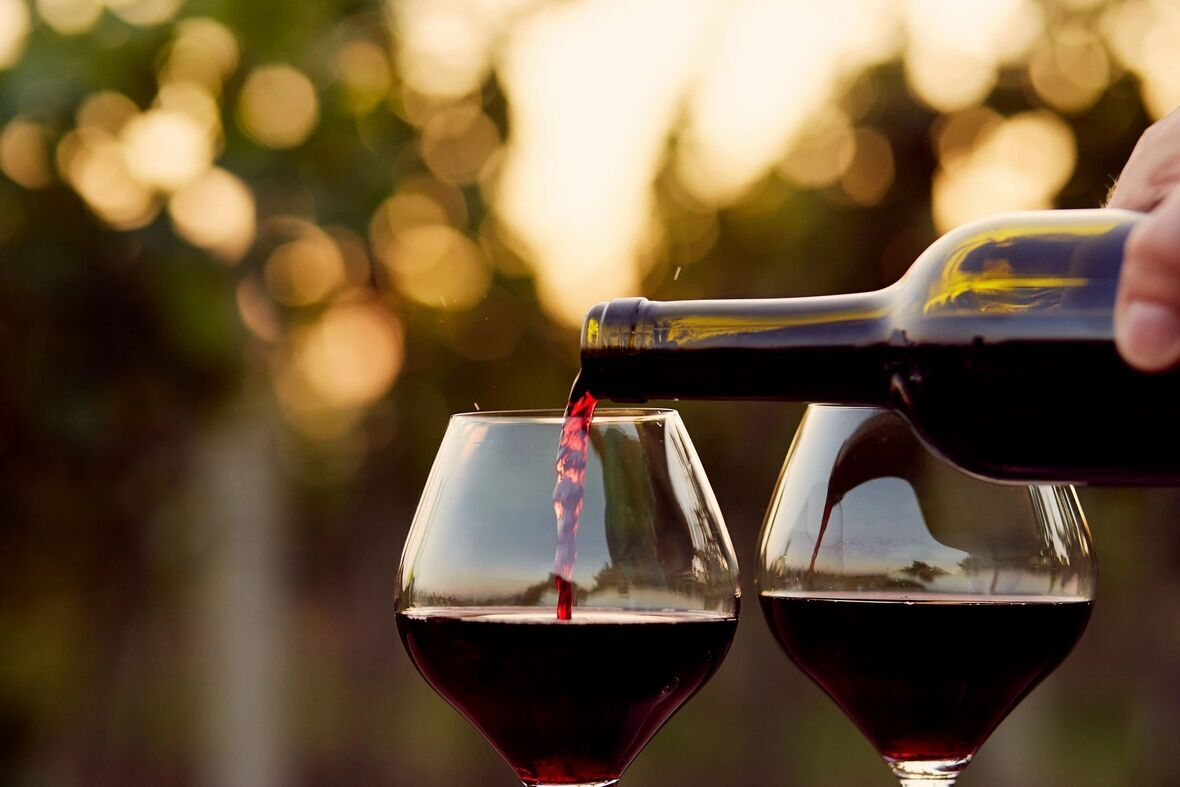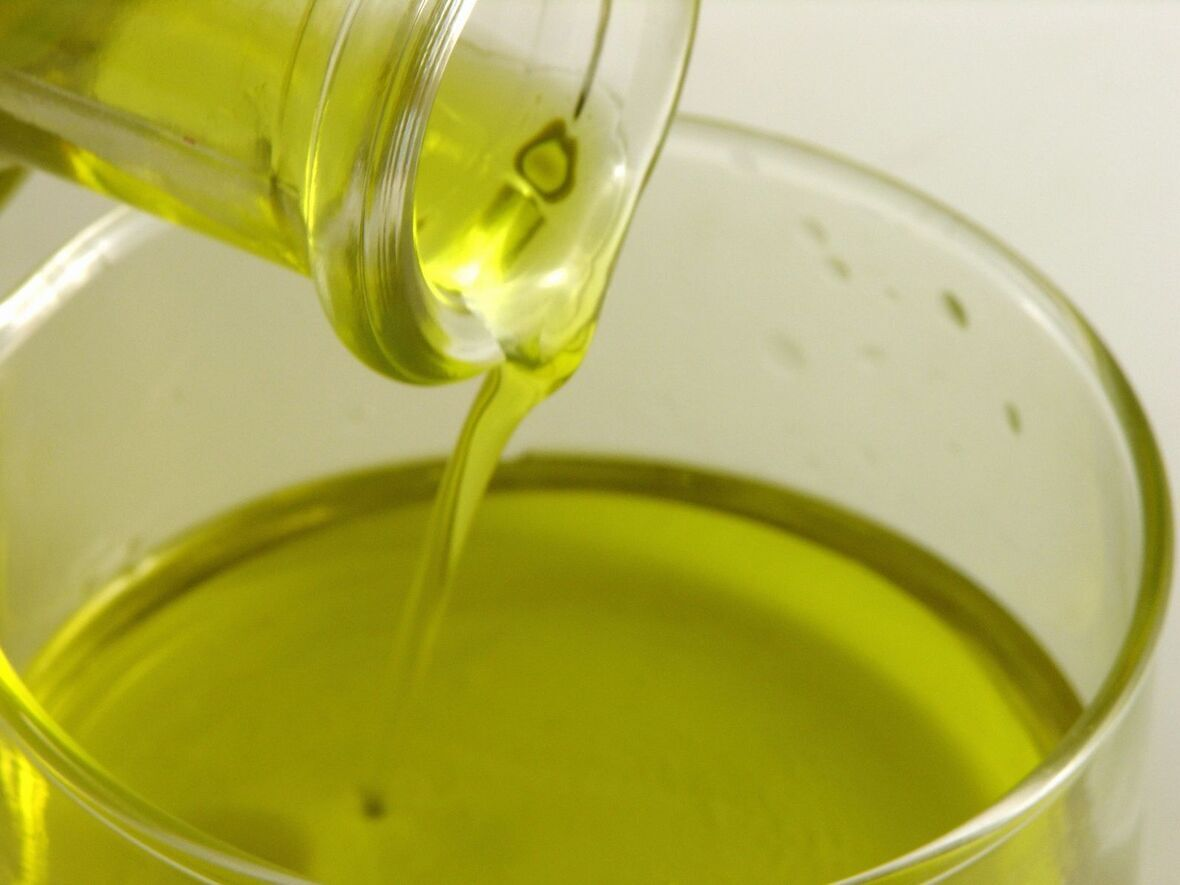# This is Not Your Average Zoom Call!

 /* styles */ This event is FREE to ALL Shall We Wine Members. The cost is \$15 for non-members.
 table div table+table+table+table+table+table+table div table{width:100%;padding:0}table div table+table+table+table+table+table+table div table img{width:96.23%;padding:0;float:none}table div table+table+table+table+table+table+table div table td{width:100%;padding:0 1.88% 18px}/* styles */## LEARNLearn about natural wines, orange wines and olive oils from Tuscany!

 table div table+table+table+table+table+table+table+table+table+table div table{width:100%;padding:0}table div table+table+table+table+table+table+table+table+table+table div table img{width:96.23%;padding:0;float:none}table div table+table+table+table+table+table+table+table+table+table div table td{width:100%;padding:0 1.88% 18px}/* styles */## DRINK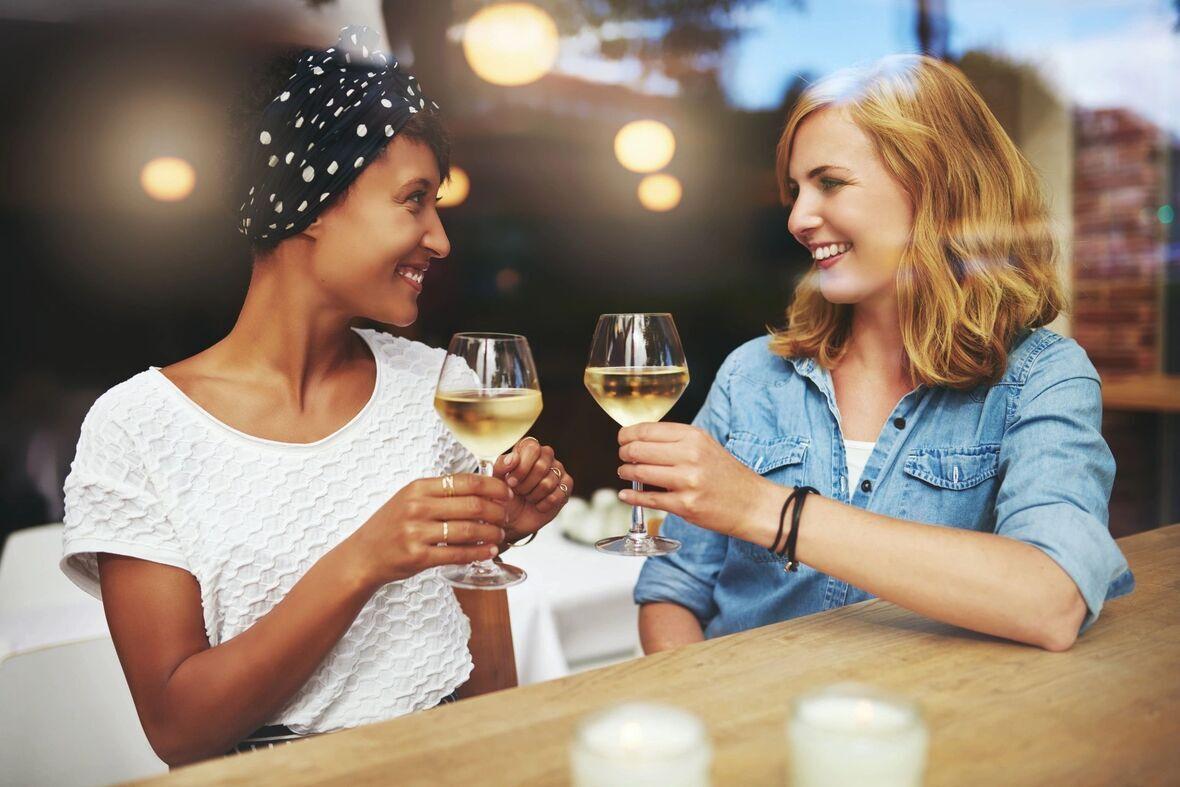Drink with our tribe and meet new friends

Purchase your bottles from our featured guests (click links below to purchase)

ORANGE WINE - La Maliosa’s natural Saturnia Bianco

NATURAL WINE - La Maliosa Saturnia Rosso 2019

ORGANIC EXTRA VIRGIN OLIVE OIL - tuscan blend, Aurinia.

 table div table+table+table+table+table+table+table+table+table+table+table+table+table div table{width:100%;padding:0}table div table+table+table+table+table+table+table+table+table+table+table+table+table div table img{width:96.23%;padding:0;float:none}table div table+table+table+table+table+table+table+table+table+table+table+table+table div table td{width:100%;padding:0 1.88% 18px}/* styles */## Tuscan Natural Wine & Olive Oil Explorer Set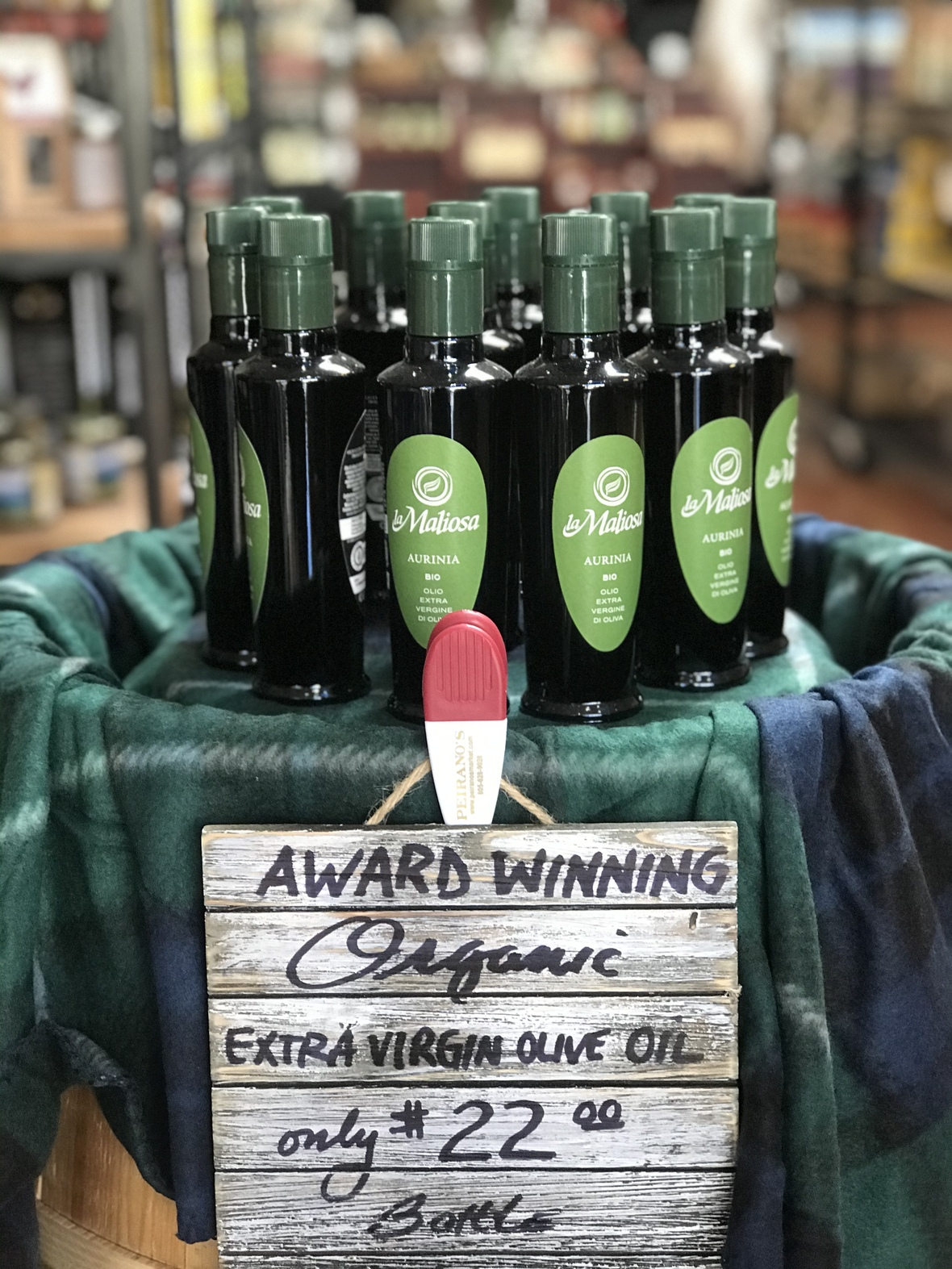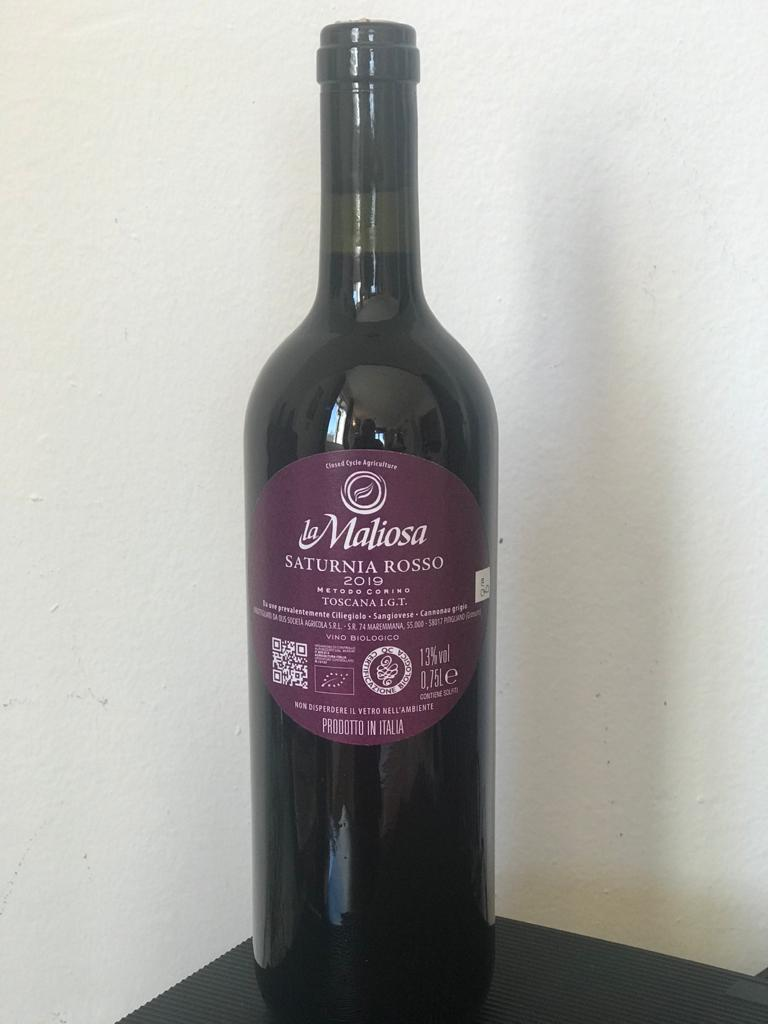Explorer Set includes:
1) A natural red wine, La Maliosa Saturnia Rosso 2019, FIRST TIME IN THE US MARKET!
2) An organic extra virgin olive oil Tuscan blend, Aurinia. The 2020 harvest is also just making it to the US

# Purchase the set for \$64.99 with free shipping here

Discount code
Shall We Wine guests get 10% off your first purchase with discount code VEROSHALLWEWINE used in our online SHOP

 table div table+table+table+table+table+table+table+table+table+table+table+table+table+table+table+table+table div table{width:100%;padding:0}table div table+table+table+table+table+table+table+table+table+table+table+table+table+table+table+table+table div table img{width:96.23%;padding:0;float:none}table div table+table+table+table+table+table+table+table+table+table+table+table+table+table+table+table+table div table td{width:100%;padding:0 1.88% 18px}/* styles */## Featured Wine - ORANGE WINE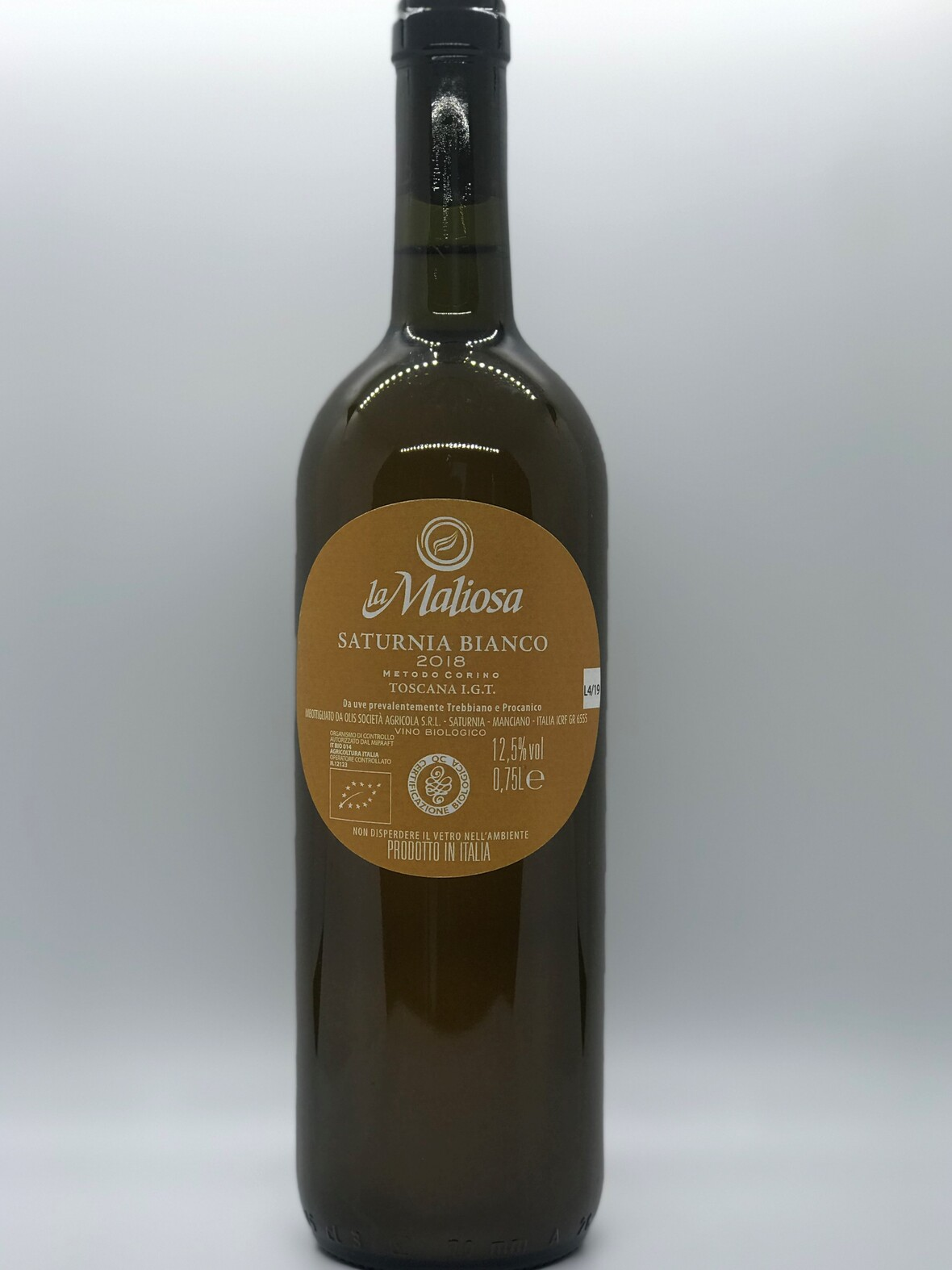La Maliosa Saturnia Bianco
 /* styles */ We will feature La Maliosa’s natural orange wine, Saturnia Bianco. People who buy the Wine and Olive Oil set above can add it on to their order to receive free shipping. La Maliosa has 2 vintages, CLICK THE LINK TO PURCHASE 2018 costing \$27.99 and 2019 costing \$29.99.
 table div table+table+table+table+table+table+table+table+table+table+table+table+table+table+table+table+table+table+table+table+table div table{width:100%;padding:0}table div table+table+table+table+table+table+table+table+table+table+table+table+table+table+table+table+table+table+table+table+table div table img{width:96.23%;padding:0;float:none}table div table+table+table+table+table+table+table+table+table+table+table+table+table+table+table+table+table+table+table+table+table div table td{width:100%;padding:0 1.88% 18px}/* styles */## PREPARING FOR THE EVENT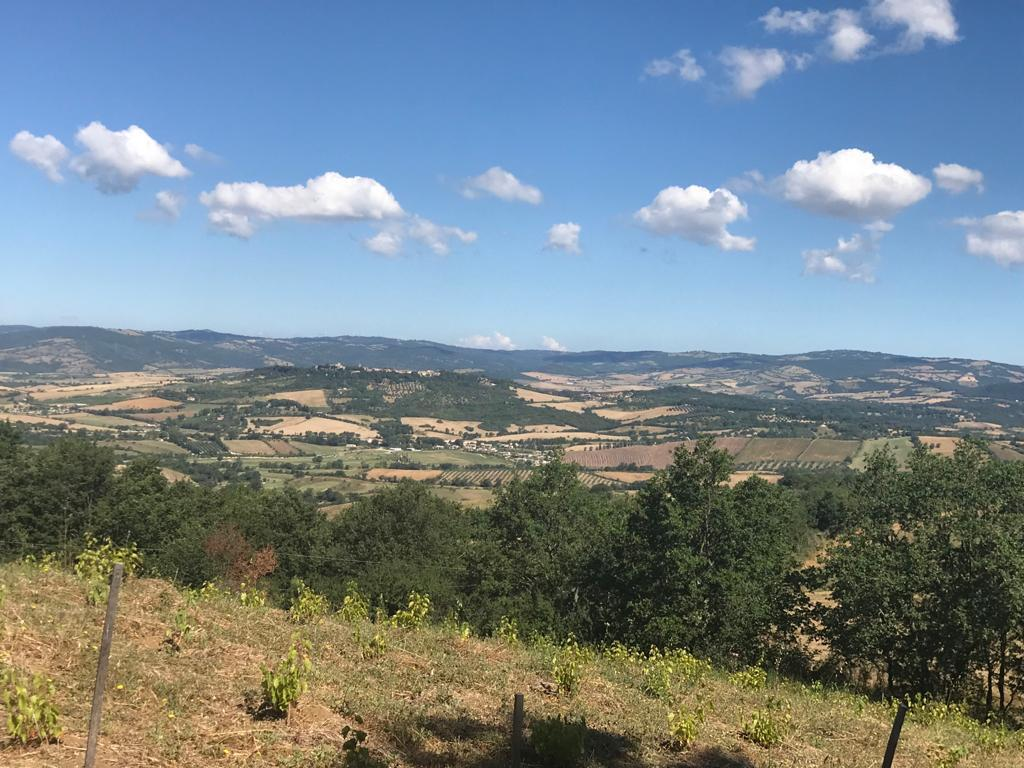Maremma Landscape from La Maliosa Vineyards

Feel free to join us in tasting the featured wine and olive oils. We will taste and discuss the La Maliosa Saturnia Rosso 2019, La Maliosa’s natural orange wine, Saturnia Bianco and Aurinia Tuscan extra virgin olive oil.

https://www.verovinogusto.com/shop
https://www.verovinogusto.com/la-maliosa

 table div table+table+table+table+table+table+table+table+table+table+table+table+table+table+table+table+table+table+table+table+table+table+table+table div table{width:100%;padding:0}table div table+table+table+table+table+table+table+table+table+table+table+table+table+table+table+table+table+table+table+table+table+table+table+table div table img{width:96.23%;padding:0;float:none}table div table+table+table+table+table+table+table+table+table+table+table+table+table+table+table+table+table+table+table+table+table+table+table+table div table td{width:100%;padding:0 1.88% 18px}/* styles */table div table+table+table+table+table+table+table+table+table+table+table+table+table+table+table+table+table+table+table+table+table+table+table+table+table+table+table div table{width:100%;padding:0}table div table+table+table+table+table+table+table+table+table+table+table+table+table+table+table+table+table+table+table+table+table+table+table+table+table+table+table div table img{width:96.23%;padding:0;float:none}table div table+table+table+table+table+table+table+table+table+table+table+table+table+table+table+table+table+table+table+table+table+table+table+table+table+table+table div table td{width:100%;padding:0 1.88% 18px}/* styles */